### 技术支持

#### 影响支承承载能力的四个参数

 静态选型1 ）选型计算流程图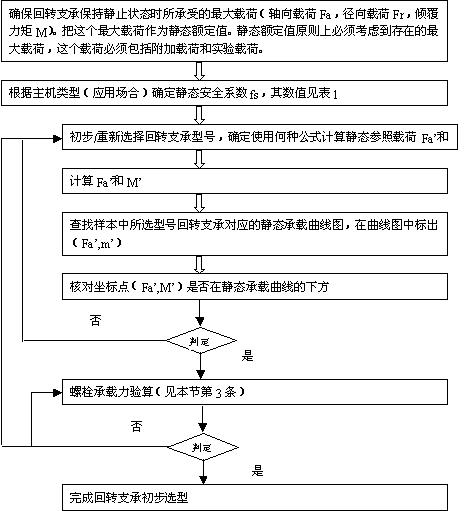2 ）静态参照载荷 Fa' 和 M' 的计算方法：·单排四点接触球式：单排四点接触球式回转支承的选型计算分别按承载角 45 ° 和 60 °两种情况进行。I、a=45° II、a=60°Fa'=(1.225*Fa+2.676*Fr)*fs Fa'=(Fa+5.046*Fr)*fsM'=1.225*M*fs M'=M*fs然后在曲线图上找出以上二点，其中一点在曲线以下即可。·单排交叉滚柱式Fa'=(Fa+2.05Fr)*fsM'=M*fs·双排异径球式对于双排异径球式回转支承选型计算，但 Fr ≦ 10%Fa 时， Fr 忽略不计。当 Fr ≧ 10%Fa 时，必须考虑轨道内侧压力角的变化，其计算请与我们联系。Fa'=Fa*fsM'=M*fs·三排滚柱式三排滚柱式回转支承选型时，仅对轴向滚道负荷和倾覆力矩的作用进行计算。Fa'=Fa*fsM'=M*fs·动态选型对于连续运转、高速回转和其它对回转支承的寿命有具体要求的应用场合，请与我公司联系。·螺栓承载力验算：·把回转支承所承受的最大载荷（没有乘静态安全系数 fs ）作为选择螺栓的载荷。·查对载荷是否在所需等级螺栓极限负荷曲线以下；·若螺栓承载能力不够，可重新选择回转支承，或与我公司联系。·选型计算距离 - 门坐式起重机（抓斗）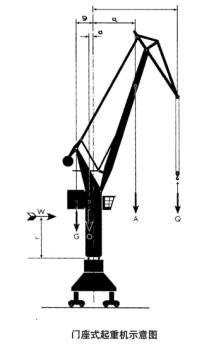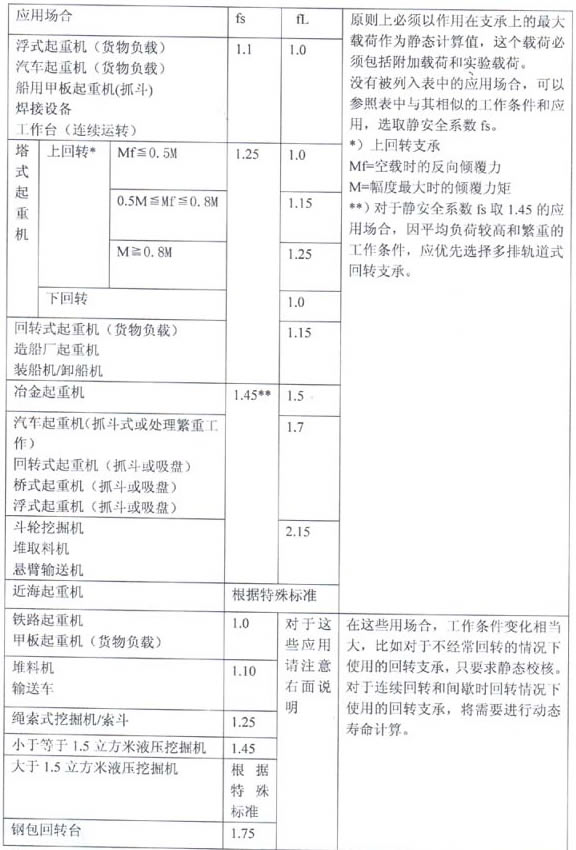·计八级风力的最大工作载荷

2) 不计风力，考虑 25% 实验负荷的载荷

Q=260KN lmax=23m

A=75KN amax=11m

O=450KN o=0.75m

G=900KN g=3m

W=27KN r=6.5m

·八级风力时的最大工作载荷：

Fa=Q+A+O+G=260+75+450+900=1685KN

M=Q*lmax+A*amax+W*r-O*o-G*g=260*23+75*11+27*6.5-450*0.75-900*3=3943KNm

2) 不计风力，考虑 25% 试验负载时的最大工作载荷

Fa=1.25Q+A+O+G=325+75+450+900=1750KN

M=1.25Q*lmax+A*amax-O*o-G*g=325*23+75*11-45*0.75-900*3=5566.3KNm

·不计风力时最大工作载荷

Fa=1685KN

M=Q*lmax+A*amax-O*o-G*g=260*23+75*11-450*0.75-900*3=3767.5KNm

Fa'=1750KN*1.45=2537.5KN

M'=5566.3KNm*1.45=8071.1KNm

Fa=1750KN

M=5566.3KNM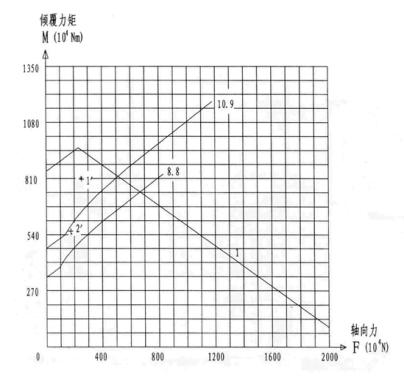l 线为静态承载能力曲线

8.8 、 10.9 为螺栓承载曲线

1'- 静态载荷点

2'- 螺栓载荷点

1' 点再滚道静态承载曲线 1 下方，因此满足要求

2' 点在 10.9 级螺栓承载曲线下方，因此选择 0.9 级螺栓可以满足要求。

0 0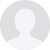# 管理员### 0516-87769952X（点击微信号复制，添加好友）